# Class 10 Science Chapter 13 Magnetic Effects of Electric Current

NCERT Solutions For Class 10 Science Chapter 13 Magnetic Effects of Electric Current.

Question 1.

Why does a compass needle get deflected when brought near a bar magnet?
This is because of magnetic field of the bar magnet.

Question 2.
Draw magnetic field lines around a bar magnet.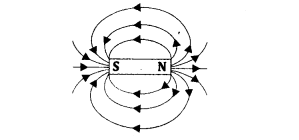Question 3.
List the properties of magnetic lines of forces.
Properties of Magnetic Lines of Force

1. Magnetic field lines are closed and continuous curves.
2. These lines always begin from the north pole of a magnet and merge into the south pole forming closed curves outside the magnet.
3. Inside the magnet, these lines of force originate from its south pole and go towards the north pole.
4. These lines are crowded near the poles where the magnetic field is strong and are far apart where the magnetic field is weak.
5. These lines never intersect each other.Question 4.
Why do not two magnetic lines of forces intersect each other?
If the magnetic lines of forces intersect, there will be two different directions of magnetic field at that point, which is not possible.

Question 5.
Consider a circular loop of wire lying in the plane of the table. Let the current passes through the loop clockwise. Apply the right- hand rule to find out the direction of the magnetic field inside and outside the loop.
(a) Direction of magnetic field inside the loop is perpendicular to the plane of the paper inward (anticlockwise).
(b) Direction of magnetic field outside the loop is perpendicular to the plane of the paper outward (clockwise).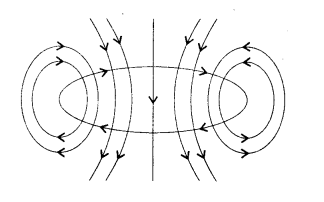Question 6.
The magnetic field in a given region is uniform. Draw a diagram to represent it.
As shown in the figure the parallel and equidistant magnetic field lines representing a uniform magnetic field in.a region.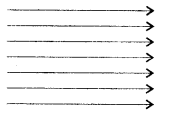Question 7.
Choose the correct option. The magnetic field inside a long straight solenoid carrying current
(a) is zero.
(b) decreases as we move towards its end.
(c) increases as we move towards its end.
(d) is the same at all points.
(d) is the same at all points.

Question 8.
Which of the following properties of a proton can change while it moves freely in a magnetic field? (There may be more than one’ correct answer.)
(a) Mass
(b) Speed
(c) Velocity
(d) Momentum
(c) Velocity and  (d) Momentum

Question 9.
In activity 13.7 .of NCERT, how do we think the displacement of rod AB will be affected if
(a) current in rod AB is increased
(b) a stronger horse-shoe magnet is used; and
(c) length of the rod AB is increased?
(a) Displacement of rod AB will increase on increasing the current.
(b) Displacement of rod AB will increase if a stronger horse-shoe magnet is used
(c) Displacement of rod AB will increase because the force acting on rod will increase on increasing the length of it

Question 10.
A positively-charged particle (alpha-particle) projected towards west is deflected towards north by a magnetic field. The direction of magneticfield is
(a) towards south
(b) towards east
(c) downward
(d) upwards
(d) upwards

Question 11.
State Fleming’s left-hand rule.
Fleming’s Left-hand Rule: According to this rule, stretch the forefinger, middle finger and thumb of your left hand such that they are mutually perpendicular to each other. If the forefinger and the middle finger point in the direction of magnetic field and the current respectively, then the thumb will point in the direction of motion or the force acting on the conductor.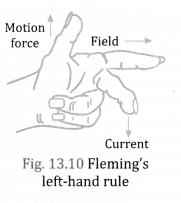F = BIL sin θ
Where, F = Force on the conductor, B = magnitude of magnetic field, I – current passing through the conductor, L = length of the conductor inside the magnetic field and 9 = angle between the conductor and the magnetic field.
Also F= Bqv
Where, q = amount of charge passing through the conductor and v = velocity of charge passing through the conductor.

Question 12.
What is the principle of an electric motor?
An electric motor works on the principle that when a current-carrying coil is placed in a magnetic field, it experiences a torque which tends to rotate it.

Question 13.
Name two safety measures commonly used in electric circuits and appliances.
(a) Earthing
(b) Electric fuse

Question 14.
An electric oven of 2 kW power rating is operated in a domestic electric circuit (220 V) that has a current rating of 5 A. What result do you expect? Explain.
Given: P = 2 kW = 2000W and V= 220 volts, I = ?
Applying the formula P = Vl, we get
I = P V = 2000 220 = 9 A
Since the current flowing (9 A) through flte circuit is more than the current rating (5 A) ofthe circuit, so the fuse in the circuit will melt and circuit is broken but electric oven is saved from getting damaged.

Question 15.
What precaution should be taken to avoid the overloading of domestic electric circuits?
We should not connect too many appliances to a single socket.

Question 16.
Explain different ways to induce current in a coil.
To induce current in a coil, there should be a:

• relative motion between the coil and a magnet.
• change in the current in a conductor placed near die coil.
• relative motion between the coil and a conductor carrying current.

Question 17.
State the principle of an electric generator.
It is based on the principle of electromagnetic induction in which a changing magnetic field in a conductor induces a current in it.

Question 18.
Name some sources of direct current.
A cell, a battery, a DC generator.

Question 19.
A rectangular coil of copper wires is rotated in a magnetic field. The direction of the induced current changes once in each
(a) two revolutions
(b) one revolution
(c) half revolution
(d) one-fourth revolution
(c) half revolution

Question 20.
Which sources produce alternating current?
An AC generator and common inverter.

Question 21.
What is the role of the split ring in an electric motor?
The function of split ring is to reverse the direction of current flowing through the coil after every half rotation of the coil.

Chapter End Questions

Question 1.
Which of the following correctly describes the magnetic field near a long straight wire?
(a) The field consists of straight lines perpendicular to the wire.
(b) The field consists of straight lines parallel to the wire.
(c) The field consists of radial lines originating from the wire.
(d) The field consists of concentric circles centred on the wire.
(d) The field consists of concentric circles centred on the wire.

Question 2.
The phenomenon of electromagnetic induction is
(a) the process of charging a body.
(b) the process of generating magnetic field due to a current passing through a coil.
(c) producing induced current in a coil due to relative motion between a magnet and the coil.
(d) the process of rotating a coil of an electric motor.
(c) producing induced current in a coil due to relative motion between a magnet and the coil.

Question 3.
The device used for producing electric current is called a
(a) generator.
(b) galvanometer.
(c) ammeter.
(d) motor.
(a) generator.

Question 4.
The essential difference between an AC generator and a DC generator is that
(a) AC generator has an electromagnet while a DC generator has permanent magnet.
(b) DC generator will generate a higher voltage.
(c) AC generator will generate a higher voltage.
(d) AC generator has slip rings while the DC generator has a commutator.
(d) AC generator has slip rings while the DC generator has a commutator.

Question 5.
At the time of short circuit, the current in the circuit
(a) reduces substantially
(b) does not change
(c) increases heavily
(d) vary continuously
(c) increases heavily

Question 6.
State whether the following statements are true or false.
(a) An electric motor converts mechanical energy into electrical energy.
(b) An electric generator v^orks on the principle of electromagnetic induction.
(c) The field at the centre of a long circular coil carrying current will be parallel straight lines.
(d) A wire with a green insulation is usually the live wire of an electric supply.
(a) False
(b) True
(c) True
(d) False

Question 7.
List three sources of magnetic fields.
Three sources of magnetic fields are:

1. Permanent magnets (like lodestone)
2. An electromagnet
3. A current-carrying conductor.

Question 8.
How does a solenoid behave like a magnet? Can you determine the north and south poles of a current-carrying solenoid with the help of a bar magnet? Explain.
When a solenoid carrying current is freely suspended, it comes to rest along a particular direction because it behaves like a bar magnet with fixed polarities at its ends.

Yes, the north and south poles of current-carrying solenoid can be determined with the help of a bar magnet

On bringing south pole of a bar magnet near one of the ends of solenoid, if the end moves towards the south pole of the bar magnet, it is north pole. But if the end moves away from the south pole of the bar magnet, it is south pole.

Question 9.
When is the force experienced by a current-carrying conductor placed in a magnetic field largest?
OR
Under what condition does a current carrying conductor kept in a magnetic field experience maximum force?
When the current-carrying conductor and magnetic field both are perpendicular to each other, the force experienced is largest.

Question 10.
Imagine that you are sitting in a chamber with your back to one wall. An electron beam, moving horizontally from back wall towards the front wall is deflected by a strong field on your right side. What is the direction of magnetic field?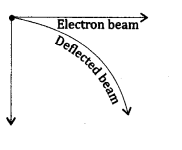According to Fleming’s left- hand rule, the direction of magnetic field will be in the downward direction perpendicular to both the directions of current and force.

Question 11.
Draw a labelled diagram of an electric motor. Explain its principle and working. What is the function of a split ring an electric motor?
An electric motor is a device which converts electrical energy into mechanical energy.
Construction: It consists of a rectangular coil called armature curved magnet pieces, commutator and carbon brushes.
Principle: It works on the principle that when a current-carrying coil is placed normally in a magnetic field, it experiences a torque which tends to rotate it.Working:

1. When a current flows through the coil, arms AB and CD experience a force. According to Fleming’s left hand rule, arm AB of the coil experiences a force in the downward direction. Similarly, arm CD of the coil experiences a force in the upward direction. Two equal and opposite forces constitute a couple and hence the coil will rotate in anticlockwise direction.
2. When the coil reaches the vertical position, no current flows in the coil and no force actstm the coil in this position.
3. Now coil rotates further and side AB is moved on the right, with its commutator segment touching the right hand brush so it has downward force acting it. Similarly, side CD has an upward force on it. Thus, the couple always acts in the same direction (clockwise) and the coil spins.

Question 12.
Name some devices in which electric motors are used. ,
Electric motors are used in fans, coolers, computers, washing machines, etc. In factories, motors are used in almost all type of machines.

Question 13.
A coil of insulated copper wire is connected to a galvanometer. What will happen if a bar magnet is:
(a) pushed into the coil?
(b) withdrawn from inside the coil?
(c) held stationary inside the coil?
(a) There will be a momentary deflection in the needle of the galvanometer towards the right This indicates that a current flows through the coil.
(b) The needle of the galvanometer deflect towards left showing that the current is set opposite to the direction of the previous flow.
(c) There will be no deflection in the needle of galvanometer.

Question 14.
Two circular coils A and B are placed closed to each other. If the current in the coil A is changed, will some current be induced in the coil B? Give reason.
When the current in the coil A is changed, the magnetic field lines connected with it also change. This induces a change in magnetic flux in the coil B. As a result of this, current is induced in the coil B.

Question 15.
State the rule to determine the direction of a
(a) magnetic field produced around a straight conductor-carrying current,
(b) force experienced by a current-carrying straight conductor placed in a magnetic field which is perpendicular to it, and
(c) current induced in a coil due to its rotation in a magnetic field.
(a)  “Right-hand thumb rule”
Right-hand Thumb Rule:
According to this rule, if the fingers of the right hand grip the wire with the thumb pointing in the direction of the current, the fingers show the direction of the magnetic field.(b) “Fleming’s left-hand rule”
Fleming’s Left-hand Rule: According to this rule, stretch the forefinger, middle finger and thumb of your left hand such that they are mutually perpendicular to each other. If the forefinger and the middle finger point in the direction of magnetic field and the current respectively, then the thumb will point in the direction of motion or the force acting on the conductor.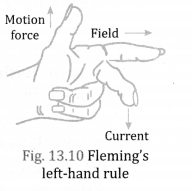F = BIL sin 6
Where, F = Force on the conductor, B = magnitude of magnetic field, I – current passing through the conductor, L = length of the conductor inside the magnetic field and 9 = angle between the conductor and the magnetic field.
Also F= Bqv
Where, q = amount of charge passing through the conductor and v = velocity of charge passing through the conductor.

(c) “Fleming’s right-hand rule”
Fleming’s Right-hand Rule: According to Fleming’s right-hand rule, stretch the forefinger, central finger and the thumb of your right Magnetk fie!d hand, mutually at right angles to each other. If the forefinger points m the direction of magnetic field and thumb denotes the direction of motion of conductor, the central finger gives the direction of flow of induced current.Question 16.
What is the function of an earth wire? Why is it necessary to earth metallic appliances?
Earth wire is used as a safety measure to prevent electric shocks in case of leakage of current to the metallic body of appliances. Earthing is essential because the person touching defective appliance (where the live wire comes in contact with the metal body due to break of Insulation or otherwise) does not get a fatal shock and the appliance is saved from being damaged.

Question 17.
When does an electric short-circuit occurs?
If live wire and neutral wire come in contact with each other accidently due to damage in insulation or fault in appliances, a large current flows through the wire because of almost zero resistance leading to burning of wires. Such a situation is called short-circuiting.

Question 18.
Explain the underlying principle and working of an electric generator by drawing a labelled diagram. What is the function of brushes?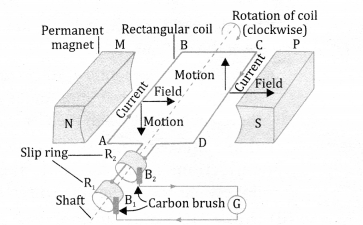Working: